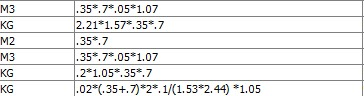Hi I have been trying to convert a column that contains equations (literally written equatios like (1*.5(8+1) in string format) but have not found any way to do it yet. It is an import from excel that is exported from a cloud software called OPUS. The workflow should run everyday so editing the excel so it calculates the formulas before importing to KNIME is not an answer. Can anyone help please?Hi,
you can use a Math Formula node to evaluate formulas. To evaluate formulas from a table, use the Table Row To Variable Loop Start and feed the formula into a Math Formula node using flow variables.
Kind regards
Alexander

1 Like

I have tryied this but the formula column is a “string” which can not be feed to a Math Formula node, and because the cells are not numbers they cant be converted from string to numbers. When I connect the variable to the Math Formula node it wont let me use any variable.

Hi,
you might have to create a dummy table. Please see attached workflow for an example.
Kind regards
Alexander
formula to variable.knwf (13.3 KB)

3 Likes

Problem solved, thank you

2 Likes

This topic was automatically closed 7 days after the last reply. New replies are no longer allowed.